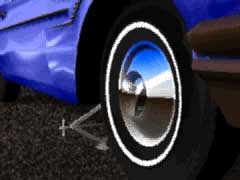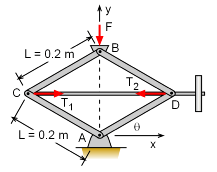Ch 10. Virtual Work & Potential Energy Multimedia Engineering Statics VirtualWork Potential Energy
 Chapter 1. Basics 2. Vectors 3. Forces 4. Moments 5. Rigid Bodies 6. Structures 7. Centroids/Inertia 8. Internal Loads 9. Friction 10. Work & Energy Appendix Basic Math Units Sections Search eBooks Dynamics Fluids Math Mechanics Statics Thermodynamics Author(s): Kurt Gramoll ©Kurt GramollSTATICS - CASE STUDY IntroductionProblem Graphic A car jack is constructed with four pinned members and a threaded shaft. The shaft must be designed to withstand the tension that results when hoisting a heavy automobile. What is known: The members of the jack are pinned together at points A, B, C, and D. The length of each member is 0.2 m. The weight of the automobile exerts a force of 1,960 N on the jack. The threaded shaft is pinned to the jack at points C and D. Under normal conditions, the jack is designed to support weight when the bottom members are at an angle between θ = 30° and θ = 60°. QuestionsForce Diagram At what angle θ is the tension in the shaft greatest under normal conditions (30o ≤ θ ≤ 60o)? At what angle θ is the tension in the shaft least? Approach Determine the virtual work performed by the weight of the automobile and the tension in the shaft. Knowing that the total virtual work must be equal to zero, derive an equation for the tension T as a function of the angle θ. Use graphic analysis to determine the maximum and minimum values of T under normal operating conditions.

Practice Homework and Test problems now available in the 'Eng Statics' mobile app
Includes over 500 problems with complete detailed solutions.
Available now at the Google Play Store and Apple App Store.